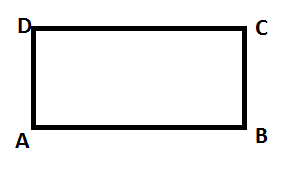Courses
Courses for Kids
Free study material
Free LIVE classes
MoreLIVE
Join Vedantu’s FREE Mastercalss

# The length, breadth and height of a room are 5m, 4m and 3m respectively. Find out the cost of white washing the walls of the room and the ceiling at the rate of Rs. 7.50 per meter square.Verified
363k+ views
Hint:
Find out the area of 4 walls and the ceiling individually. Add them together to get the total area. Then find out the costing for white wash.

Let $ABCD$ is the floor of a room. The length is $AB=CD=5m$ , breadth is $BC=CD=4m$ .
Height is 3m.Now we have to find out the area of 4 walls.
Let us first find out the area of the wall which is perpendicular to the side $AB$.
For this wall the length is 5 meter, and the breadth is the height which is 3 meter.

We know that the area of a rectangle is = $length\times breadth$
So, the area of the wall which is perpendicular to the side $AB$ is:
\begin{align} &=\left( 5\times 3 \right){{m}^{2}} \\ &=15{{m}^{2}} \\ \end{align}

The area of the wall which is perpendicular to the side $CD$ is basically the same as the area of the wall which is perpendicular to the side$AB$ . The length and the breadth, which is basically the height, are the same for both the walls.

Now the area of the wall which is perpendicular to the side $BC$ is:
$=\left( 4\times 3 \right){{m}^{2}}$
As the length of this wall is 4 meter and the breadth is 3 meter, which is the height.
So, the area is $=(4\times 3)=12{{m}^{2}}$

Similarly, the area of the wall which is perpendicular to the side $AD$ is basically the same as the area of the wall which is perpendicular to the side $BC$ . The length and the breadth, which is basically the height, are the same for both the walls.

Now, the total area of 4 walls is:
$=\left( 2\times 15 \right)+\left( 2\times 12 \right)=30+24=54{{m}^{2}}$
The area of the ceiling is the same as the floor.
So, the area of the ceiling is:
$=\left( 5\times 4 \right){{m}^{2}}=20{{m}^{2}}$

The total area is for white washing = total area of 4 walls + area of ceiling.
\begin{align} &=\left( 54+20 \right){{m}^{2}} \\ &=74{{m}^{2}} \\ \end{align}

Now, the cost of white washing for one square meter is Rs. 7.50
The cost of white washing for 74 square meters is Rs.
\begin{align} &=74\times 7.50 \\ &=555 \\ \end{align}
Therefore we need Rs. 555 to white wash the walls of the room and the ceiling.

Note: Alternatively we can directly use the area of four walls:
$=2\times \left( l+b \right)\times h$ , where $l$ is the length, $b$ is the breadth, $h$ is the height.
If we put the values in this formula:
\begin{align} & =2\times (5+4)\times 3=2\times 9\times 3 \\ & =54{{m}^{2}} \\ \end{align}
So, the area of four walls is 54 square meters.
Last updated date: 28th Sep 2023
Total views: 363k
Views today: 5.63k